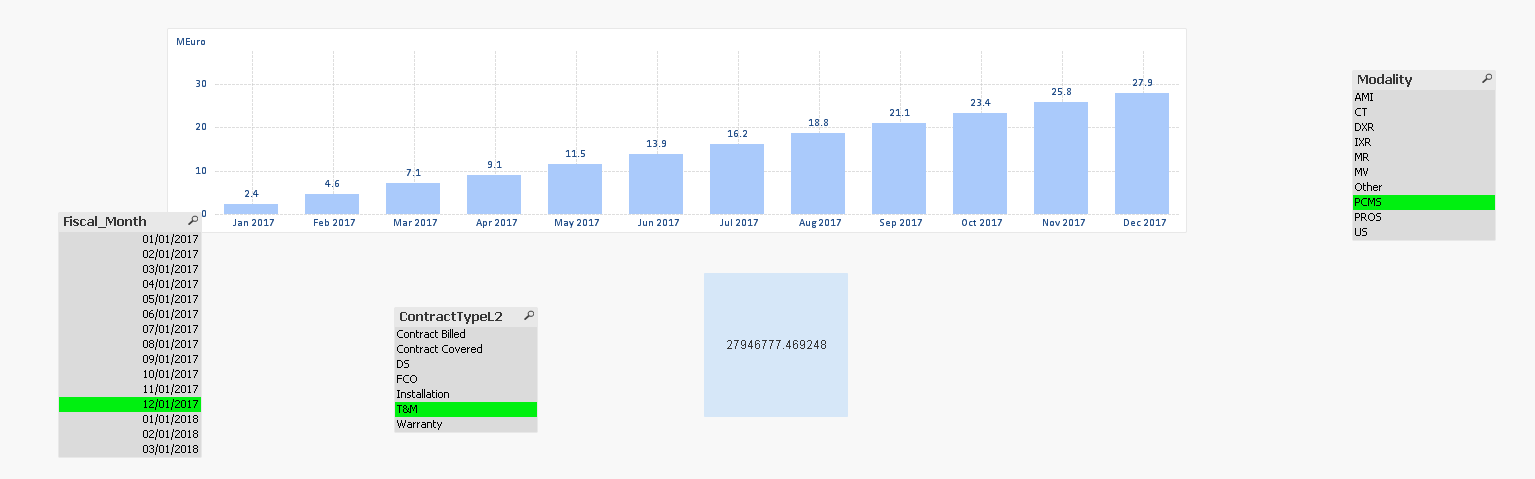# QlikView App Development

Discussion Board for collaboration related to QlikView App Development.

Contributor III

## set analysis

Hi All,

I have a modality field where it has AMI,CT,IXR,DXR,MV,MR,PCMS,PROS,US,Other.

Now i have a bar chart and i have written the below expression:

=IF (wildMatch(GetFieldSelections(Modality), '*PCMS*','*ALL*','*NOT*') AND NOT Match(GetFieldSelections(Modality), 'NOT PCMS'),

(SUM({<Fiscal_Month={">=\$(=Date(AddMonths(Max(Fiscal_Month), -11)))<=\$(=Date(Max(Fiscal_Month)))"} , Counter = {\$(v_Min_Counter)},

Modality = {'PCMS'},ContractTypeL2 ={\$(=concat(DISTINCT chr(39) & ContractTypeL2 & chr(39),','))}>}Adj_Cons_YTD_LY) )

,0)

+

(SUM({<Fiscal_Month={">=\$(=Date(AddMonths(Max(Fiscal_Month), -11)))<=\$(=Date(Max(Fiscal_Month)))"} , Counter = {96},

Modality = {\$(=concat(DISTINCT chr(39) & Modality & chr(39),','))},ContractTypeL2 ={\$(=concat(DISTINCT chr(39) & ContractTypeL2 & chr(39),','))}>}Adj_Cons_YTD_LY) )

The expression is working fine for all modalities except for PCMS . If i select PCMS the value is coming wrong.

attached qvw for more reference.

Thanks,

Bharat

1 Solution

Accepted SolutionsMVP

## Re: set analysis

I think you had an extra piece in set analysis

, Counter = {\$(v_Min_Counter)}

I removed that and it seems to work4 RepliesMVP

## Re: set analysis

Why are they wrong? I mean if the values are wrong, then what are the correct numbers?

Contributor III

## Re: set analysis

Thank you Sunny Bhai for reply..

For PCMS if you see in text box it is around 27thousand i should get that one but i am getting as 530k.

Only if  i select pcms this is happening but if i select any other in modality the value is exactly same.MVP

## Re: set analysis

I think you had an extra piece in set analysis

, Counter = {\$(v_Min_Counter)}

I removed that and it seems to workContributor III

## Re: set analysis

Yes Sunny Bhai.. i have removed it is assigned as min of that one it is by default taking the 1 (which is the minimum)value.

Thank you sunny bhai..